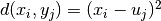# cdtw.dtw.dtw_mat¶

cdtw.dtw.dtw_mat(x, y)[source]

Computes the full cost (distance) matrix `D` between all elements of `x` and `y` according towhereis the Euclidean metric. A squared root of the output matrix `D` is returned.

Parameters
x, ynp.ndarray

Input time series, 1-d arrays of arbitrary length.

Returns
cost_matnp.ndarray

A squared root of the distance matrix `D`.